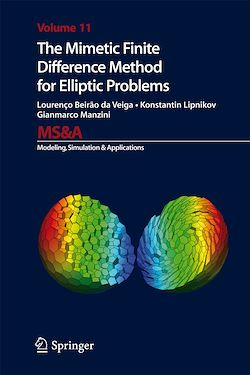# The Mimetic Finite Difference Method for Elliptic Problems

-English
394 Pages

Description

This book describes the theoretical and computational aspects of the mimetic finite difference method for a wide class of multidimensional elliptic problems, which includes diffusion, advection-diffusion, Stokes, elasticity, magnetostatics and plate bending problems. The modern mimetic discretization technology developed in part by the Authors allows one to solve these equations on unstructured polygonal, polyhedral and generalized polyhedral meshes. The book provides a practical guide for those scientists and engineers that are interested in the computational properties of the mimetic finite difference method such as the accuracy, stability, robustness, and efficiency. Many examples are provided to help the reader to understand and implement this method. This monograph also provides the essential background material and describes basic mathematical tools required to develop further the mimetic discretization technology and to extend it to various applications.

Subjects

##### Differential equation

Informations